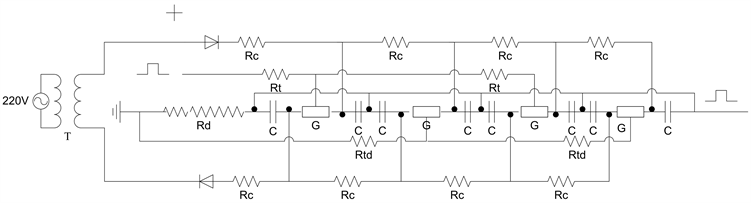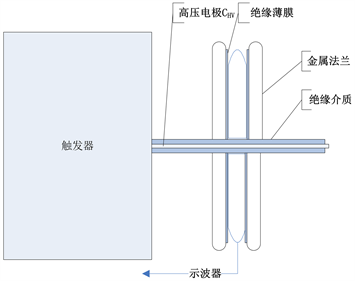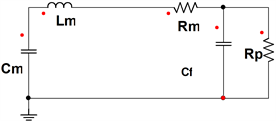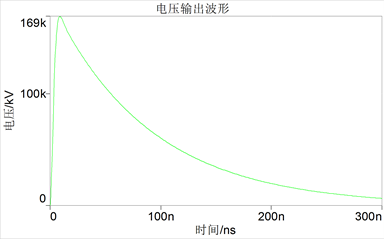﻿ 160 kV，10 ns上升沿脉冲触发器的研制

# 160 kV，10 ns上升沿脉冲触发器的研制Development of Pulsed Trigger in 160 kV and 10 ns Raise Time

Abstract: In order to study the key technology of early diagnosis of breast cancer in medical system, a trigger for the X-ray phase-contract imaging system is developed. The output pulse of the trigger is required: the peak voltage = 160 kV, the rise time ≤ 10 ns, and the pulse width ≤ 100 ns. The flip-flop consists of Marx generator, spark gap switch, capacitive voltage divider and output section. The Marx generator is composed of 8 stages of coaxial positive and negative electrode charge, each level is composed of 6 40 kV 3800 pF ceramic capacitors in parallel, and the design of compact loop low inductance is adopted; and the 4 spark gap switches use the cross stage trigger discharge. At the end of the output section, a ring capacitance divider is designed as a high voltage probe for triggers. The Simplorer simulation results show that when the actual load is blocked, the output voltage is 169 kV, the rise time is less than 10 ns, and the pulse width is less than 100 ns, which meets the design requirements.

1. 引言

2. 结构设计Figure 1. Machine drawing of Marx generator

2.1. Marx发生器

2.2. 电容分压器Figure 2. Circuit diagram of Marx generatorFigure 3. Circuit diagram of Marx generator

3. 电路模拟

${L}_{m}={L}_{sp}+{L}_{e}$

${L}_{sp}=2{l}_{sp}\mathrm{ln}\left(R/{r}_{g}\right)$

${L}_{e}=2{l}_{e}\mathrm{ln}\left(R/{r}_{e}\right)$

${R}_{m}=\frac{2}{{V}_{m}}\sqrt{\frac{P{d}^{2}}{\text{π}a}}\sqrt{\frac{{L}_{m}}{{C}^{3}}}$

$C=\frac{{C}_{m}{C}_{f}}{{C}_{m}+{C}_{f}}$

${Z}_{d}=136{\left(d/r\right)}^{2}{U}_{d}^{-1/2}$Figure 4. Circuit diagram of Marx generatorFigure 5. Circuit diagram of Marx generator

4. 总结

 叶芳, 曾蒙苏, 严福华, 等. MRI 结合数字化钼靶诊断乳腺癌的临床研究[J]. 放射学实践, 2007, 22(11): 1139-1143.

 谭辉, 张宏伟, 顾宇叁, 等. SPECT/CT和乳腺专用伽玛显像对乳腺癌诊断价值的对比研究[J]. 复旦学报, 2015, 42(6): 716-721.

 邹晓兵, 朱宏林, 曾乃工, 等. 纳秒级高压快脉冲发生器的研制[J]. 高电压技术, 2011, 37(3): 787-792.

 邹俭, 王川, 曾乃工, 等. 紧凑型Ｘ箍缩脉冲功率发生器[J]. 强激光与粒子束, 2012, 24(3): 663-667.

 杨大为, 曾乃工, 王晓军, 等. 变阻抗线及预脉冲开关的设计与调整[J]. 强激光与粒子束, 1991, 3(3): 398-404.

 邹俭, 曾乃工, 王川, 等. 天光Ⅱ-B光源的初步实验[J]. 原子能科学技术, 2013, 47(7): 1268-1271.

 邹俭, 曾乃工, 王川, 等. 天光Ⅱ-B强流脉冲电子加速器的设计[J]. 原子能科学技术, 2013, 47(5): 875-879.

 杨大为, 王乃彦, 张奎方, 等. 强流脉冲电子加速器充电调整[J]. 原子能科学技术, 1988, 22(2): 134-147.

 邹俭, 王川, 郑侠, 等. 紧凑型X-pinch装置探头标定[J]. 强激光与粒子束, 2011, 6(6): 1687-1691.

 邹俭, 曾乃工, 王川, 等. 天光Ⅱ-B装置的诊断刻度[J]. 原子能科学技术, 2011, 45(12): 1502-1505.

 曾乃工, 杨大为, 姜兴东, 等. 天光Ⅱ号强流脉冲电子加速器[C]//高电压新技术分委会. 第五届全国高功率会议论文集. 合肥: 高电压新技术分委会, 1993: 207-211.

 邹俭, 王川, 曾乃工, 等. 混合电极结构下的X-pinch研究[J]. 原子能科学技术, 2015, 49(12): 2251-2254.

 邹俭, 曾乃工, 王川, 等. 基于X-pinch丝负载的背光照相研究[J]. 原子能科学技术, 2017, 51(8): 1484-1487.

 Shelkovenko, T.A., Pikuz, S.A., Cahill, A.D., et al. (2010) Hybrid X-Pinch with Conical Electrodes. Physics of Plasmas, 17, Article ID: 112707.
https://doi.org/10.1063/1.3504226

 曾正中. 脉冲功率技术引论[M]. 西安: 陕西科学技术出版社, 2003: 147-180.

Top